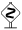# PyX — Example: text/textbox.py

0.3 KB
16.7 KB
18.9 KB
10.6 KB
7.9 KB

## Enlarge the surrounding of a textbox```from pyx import *
unit.set(xscale=3)

tbox = text.text(0, 0, r"Boxed text")
tpath = tbox.bbox().enlarged(3*unit.x_pt).path()

c = canvas.canvas()
c.draw(tpath, [deco.filled([color.cmyk.Yellow]), deco.stroked()])
c.insert(tbox)
c.writeEPSfile("textbox")
c.writePDFfile("textbox")
c.writeSVGfile("textbox")
```

### DescriptionThis example introduces the concept of the `textbox`, which is a canvas-like structure containing the text and its surrounding path and reference point. We demonstrate how to enlarge the surrounding box a little and use it as a decoration of the path.The `textbox` is a specialized version of the `box` concept which has been introduced into PyX in a preliminary version. In the example, we obtain the bounding box (this is a `bbox` instance, not to be mixed up with the `box`) and enlarge it a bit. We then draw the path of the bounding box.In future releases of PyX, the boxes will have generic support for enlarging. The syntax will then be

```tpath = tbox.enlarged(3*unit.x_pt).path()
```An alternative, geometrically different approach to enlarging the textbox path is via an appropriate transformation, such as

```tbox = text.text(0, 0, r"Boxed text", [text.halign.boxcenter, text.valign.middle])
tpath = tbox.path().transformed(trafo.scale(1.5, 1.5, 0, 0))
```